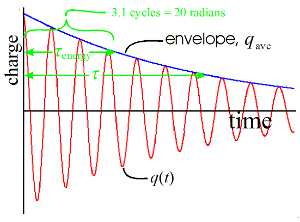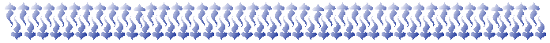There are all sorts of resonances around us, in the world, in our culture, and in our technology. A tidal resonance causes the 55 foot tides in the Bay of Fundy. Mechanical and acoustical resonances and their control are at the center of practically every musical instrument that ever existed. Even our voices and speech are based on controlling the resonances in our throat and mouth. Technology is also a heavy user of resonance. All clocks, radios, televisions, and gps navigating systems use electronic resonators at their very core. Doctors use magnetic resonance imaging or MRI to sense the resonances in atomic nuclei to map the insides of their patients. In spite of the great diversity of resonators, they all share many common properties. In this blog, we will delve into their various aspects. It is hoped that this will serve both the students and professionals who would like to understand more about resonators. I hope all will enjoy the animations.

Origins of Newton's laws of motion

History of mechanical clocks with animations
Understanding a mechanical clock with animations
includes pendulum, balance wheel, and quartz clocks

## Friday, April 1, 2011

### 2.10 Q, quality factor of a resonatorFigure 1. Decay of an LRC circuit resonator. The decay time τ is the time for the amplitude of oscillations to decay to 1/e or 37% of the original amplitude. Energy and power (not shown) decay twice as fast, i.e. τenergy = τ/2 . This graph also shows τenergy given in terms of cycles and radians: 3.1 cycles (you can count them off the graph) and 20 radians (multiply the cycles by 2π or 6.28). The Q of this resonator is 20, i.e. the decay time of energy in units of radians.

## 2.10 Q, quality factor

The Q or quality factor is used by experts of resonators to rate how good a resonator is. It is similar to the time constant but instead of seconds, it is a measure of the decay time (i.e. ring time) of a shock-excited resonator in terms of radians (i.e. 2π times the decay time in cycles). It is particularly useful for comparing resonators that resonate at different frequencies. Table 1 lists a few typical Q's.

Table 1. Typical Q's of various resonators
LRC resonant circuits 10 - 1000
balance wheel 100 - 300
tuning fork 1000
pendulum clock 3000 - 100,000
quartz crystals in watches 10,000 - 1,000,000

### Definition

The time constant and Q are related by:

Q = ω0τenergy    ,     (1)

where ω0 is the angular resonant frequency of the resonator and τenergy is the resonator's time constant for energy decay.

We illustrate this in Figure 1, at right. The particular resonator graphed has an energy decay time in cycles of 3.1 cycles or 20 radians. The 20 radians is the Q of this resonator. To further understand this, we can consider Equation (1) to be a unit conversion as:

Radians is a non-unit and is usually ignored, unless it is particularly illuminating to call it out. Thus Q is considered to be without units, i.e. a pure number.

The Q of a resonator is the number of radians required for the resonator's energy to decay to 1/e or 37% of its initial energy when the resonator is shock excited.### Power loss

The power loss of a decaying resonator can also be related to its Q. Using Equation (a) of Section 2.6 and Equation (1) above, we get

We see that power loss is inversely proportional to the Q. The higher the Q, the less power is lost. The equation works the other way also, the more power lost, the lower the Q. This means that even if the resonator has very low internal losses (such as those due to friction), if we couple an outside device to it that takes power from it so that its power loss is large, then its Q will be lower. High Q requires both low internal losses and low coupling losses, i.e. weak coupling to the outside.Figure 2. Resonance curve. Energy in a resonator versus the frequency that is powering it (in a constant powering situation). High Q resonators have narrow resonance curves and as a consequence are the critical element in precise timing and frequency selection devices and circuits.

### Bandwidth

In the next chapter we will discuss a resonance curve and bandwidth in detail, however a very short summary is in order here. Figure 2 shows the energy in a resonator that is continuously driven by a sinusoidal source as a function of the frequency of that source. The peak occurs at the resonant frequency f0. The bandwidth or width of the curve half way up is Δf½ (in units of Hz, i.e. cycles per second). The resonant frequency and bandwidth in units of radians per second are denoted by ω0 and Δω½. These are related to Q by:Thus we see that the bandwidth is inversely proportional to the Q of a resonator; that the higher the Q, the smaller the bandwidth and the narrower the resonance curve.

P. Ceperley Feb. 2010